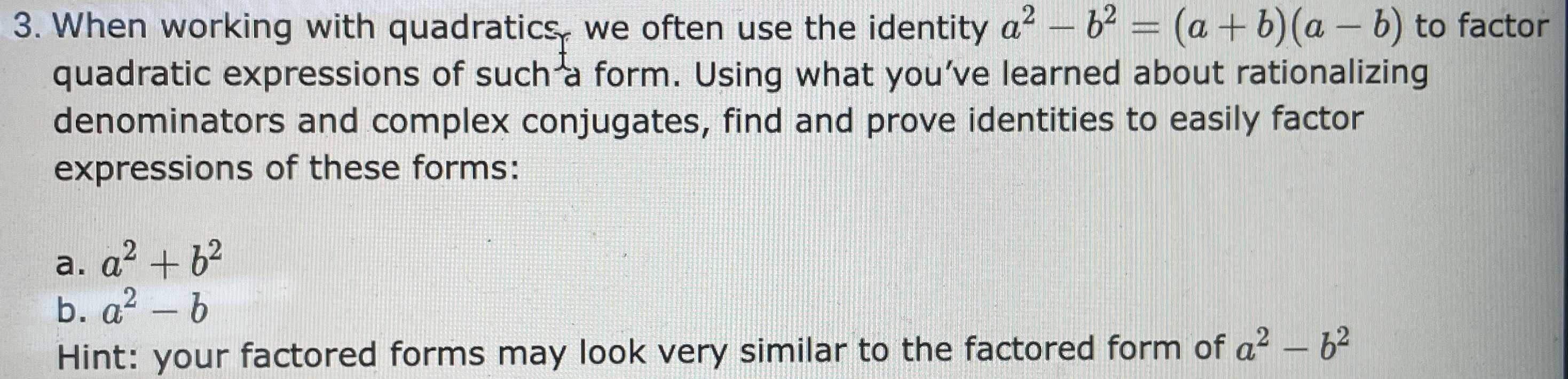### ¿Todavía tienes preguntas de matemáticas?

Pregunte a nuestros tutores expertos
Algebra
Pregunta3. When working with quadratics, we often use the identity $$a ^ { 2 } - b ^ { 2 } = ( a + b ) ( a - b )$$ to factor quadratic expressions of such $$_ { a }$$ form. Using what you've learned about rationalizing denominators and complex conjugates, find and prove identities to easily factor expressions of these forms:

a. $$a ^ { 2 } + b ^ { 2 }$$

b. $$a ^ { 2 } - b$$

Hint: your factored forms may look very similar to the factored form of $$a ^ { 2 } - b ^ { 2 }$$

(a) $$a^2+ b^2 = (a + b + \sqrt{2ab} )(a + b - \sqrt{2ab})$$ or $$a^2 - b^2 = (a+ ib)(a- ib)$$
(b) $$a^2 - b^2 = (a+ b)(a- b)$$